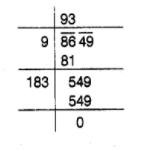# 8649 students were sitting in a lecture room

Question:

8649 students were sitting in a lecture room in such a manner that there were as many students in the row as there were rows in the lecture

room. How many students were there in each row of the lecture room?

Solution:

Let number of students in each row of the lecture room be $x$. Then, number of rows $=x$$\therefore$ Total students $=x \times x=x^{2}$

According to the question,

$x^{2}=8649$

$\Rightarrow \quad x=\sqrt{8649}$

$\therefore \quad x=93$

Hence, there are 93 students in each row of the lecture room.